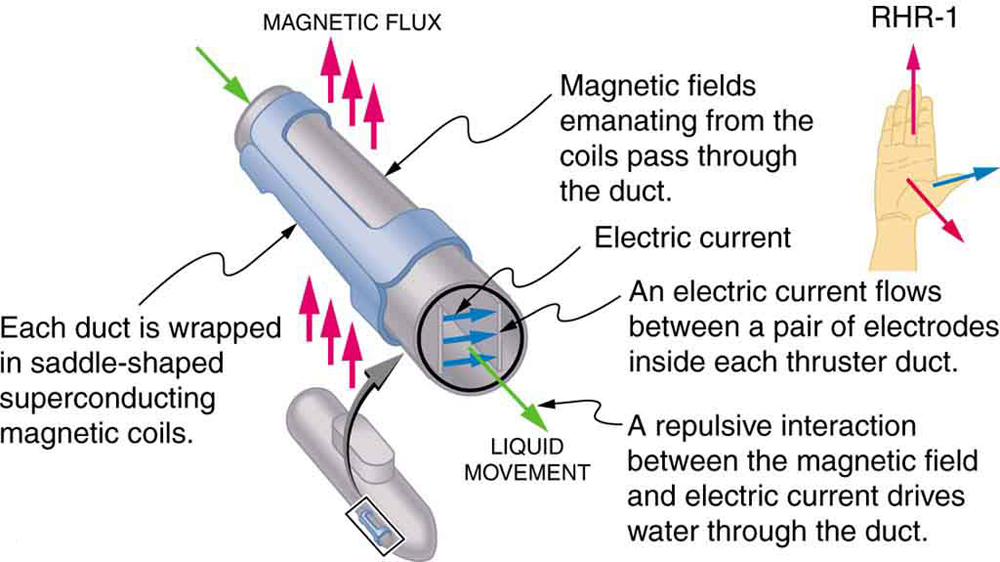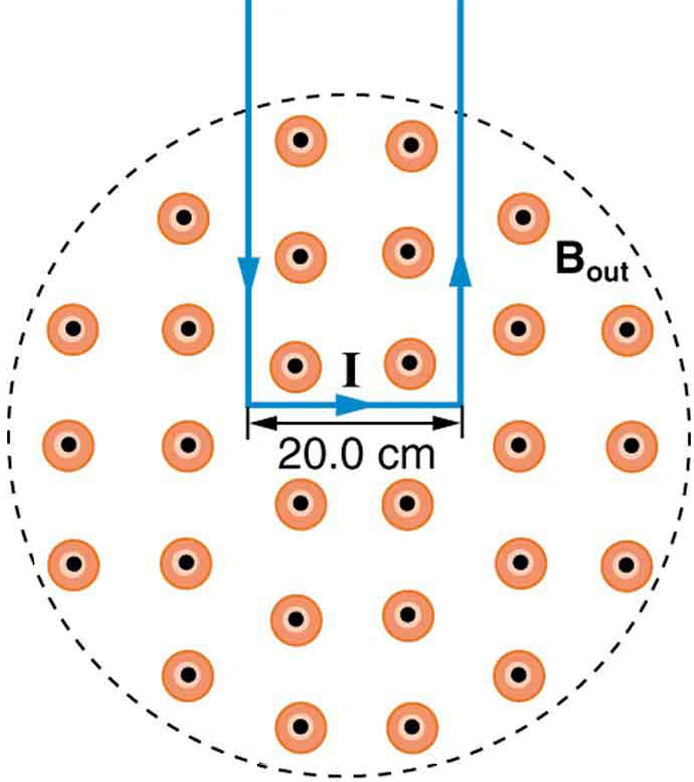# 22.7 Magnetic force on a current-carrying conductor  (Page 2/2)

 Page 2 / 2An MHD propulsion system in a nuclear submarine could produce significantly less turbulence than propellers and allow it to run more silently. The development of a silent drive submarine was dramatized in the book and the film The Hunt for Red October .

## Section summary

• The magnetic force on current-carrying conductors is given by
$F=\text{IlB}\phantom{\rule{0.25em}{0ex}}\text{sin}\phantom{\rule{0.25em}{0ex}}\mathrm{\theta ,}$
where $I$ is the current, $l$ is the length of a straight conductor in a uniform magnetic field $B$ , and $\theta$ is the angle between $I$ and $B$ . The force follows RHR-1 with the thumb in the direction of $I$ .

## Conceptual questions

Draw a sketch of the situation in [link] showing the direction of electrons carrying the current, and use RHR-1 to verify the direction of the force on the wire.

Verify that the direction of the force in an MHD drive, such as that in [link] , does not depend on the sign of the charges carrying the current across the fluid.

Why would a magnetohydrodynamic drive work better in ocean water than in fresh water? Also, why would superconducting magnets be desirable?

Which is more likely to interfere with compass readings, AC current in your refrigerator or DC current when you start your car? Explain.

## Problems&Exercises

What is the direction of the magnetic force on the current in each of the six cases in [link] ?

(a) west (left)

(b) into page

(c) north (up)

(d) no force

(e) east (right)

(f) south (down)

What is the direction of a current that experiences the magnetic force shown in each of the three cases in [link] , assuming the current runs perpendicular to $B$ ?

What is the direction of the magnetic field that produces the magnetic force shown on the currents in each of the three cases in [link] , assuming $\mathbf{\text{B}}$ is perpendicular to $\mathbf{\text{I}}$ ?

(a) into page

(b) west (left)

(c) out of page

(a) What is the force per meter on a lightning bolt at the equator that carries 20,000 A perpendicular to the Earth’s $3\text{.}\text{00}×{\text{10}}^{-5}\text{-T}$ field? (b) What is the direction of the force if the current is straight up and the Earth’s field direction is due north, parallel to the ground?

(a) A DC power line for a light-rail system carries 1000 A at an angle of $\text{30.0º}$ to the Earth’s $\text{5.00}×{\text{10}}^{-5}\phantom{\rule{0.25em}{0ex}}\text{-T}$ field. What is the force on a 100-m section of this line? (b) Discuss practical concerns this presents, if any.

(a) 2.50 N

(b) This is about half a pound of force per 100 m of wire, which is much less than the weight of the wire itself. Therefore, it does not cause any special concerns.

What force is exerted on the water in an MHD drive utilizing a 25.0-cm-diameter tube, if 100-A current is passed across the tube that is perpendicular to a 2.00-T magnetic field? (The relatively small size of this force indicates the need for very large currents and magnetic fields to make practical MHD drives.)

A wire carrying a 30.0-A current passes between the poles of a strong magnet that is perpendicular to its field and experiences a 2.16-N force on the 4.00 cm of wire in the field. What is the average field strength?

1.80 T

(a) A 0.750-m-long section of cable carrying current to a car starter motor makes an angle of $\text{60º}$ with the Earth’s $5\text{.}\text{50}×{\text{10}}^{-5}\phantom{\rule{0.25em}{0ex}}\text{T}$ field. What is the current when the wire experiences a force of $\text{7.00}×{\text{10}}^{-3}\phantom{\rule{0.25em}{0ex}}N$ ? (b) If you run the wire between the poles of a strong horseshoe magnet, subjecting 5.00 cm of it to a 1.75-T field, what force is exerted on this segment of wire?

(a) What is the angle between a wire carrying an 8.00-A current and the 1.20-T field it is in if 50.0 cm of the wire experiences a magnetic force of 2.40 N? (b) What is the force on the wire if it is rotated to make an angle of $\text{90º}$ with the field?

(a) $\text{30º}$

(b) 4.80 N

The force on the rectangular loop of wire in the magnetic field in [link] can be used to measure field strength. The field is uniform, and the plane of the loop is perpendicular to the field. (a) What is the direction of the magnetic force on the loop? Justify the claim that the forces on the sides of the loop are equal and opposite, independent of how much of the loop is in the field and do not affect the net force on the loop. (b) If a current of 5.00 A is used, what is the force per tesla on the 20.0-cm-wide loop?A rectangular loop of wire carrying a current is perpendicular to a magnetic field. The field is uniform in the region shown and is zero outside that region.

derivative of first differential equation
why static friction is greater than Kinetic friction
draw magnetic field pattern for two wire carrying current in the same direction
An American traveler in New Zealand carries a transformer to convert New Zealand’s standard 240 V to 120 V so that she can use some small appliances on her trip.
What is the ratio of turns in the primary and secondary coils of her transformer?
nkombo
How electric lines and equipotential surface are mutually perpendicular?
The potential difference between any two points on the surface is zero that implies È.Ŕ=0, Where R is the distance between two different points &E= Electric field intensity. From which we have cos þ =0, where þ is the angle between the directions of field and distance line, as E andR are zero. Thus
sorry..E and R are non zero...
By how much leeway (both percentage and mass) would you have in the selection of the mass of the object in the previous problem if you did not wish the new period to be greater than 2.01 s or less than 1.99 s?
what Is linear momentum
why no diagrams
where
Fayyaz
Myanmar
Pyae
hi
Iroko
hello
Abdu
Describe an experiment to determine short half life
what is science
it's a natural phenomena
Hassan
sap
Emmanuel
please can someone help me with explanations of wave
Benedine
there are seven basic type of wave radio waves, gyamma rays (nuclear energy), microwave,etc you can also search 🔍 on Google :-)
Shravasti
A 20MH coil has a resistance of 50 ohms and us connected in series with a capacitor to a 520MV supply
what is physics
it is the science which we used in our daily life
Sujitha
Physics is the branch of science that deals with the study of matter and the interactions it undergoes with energy
Junior
it is branch of science which deals with study of happening in the human life
AMIT
A 20MH coil has a resistance of 50 ohms and is connected in series with a capacitor to a 250MV supply if the circuit is to resonate at 100KHZ, Determine 1: the capacitance of the capacitor 2: the working voltage of the circuit, given that pie =3.142
Musa
Physics is the branch of science that deals with the study of matter and the interactions it undergoes with energy
Kelly
Heat is transfered by thermal contact but if it is transfered by conduction or radiation, is it possible to reach in thermal equilibrium?
Yes, It is possible by conduction if Surface is Adiabatic
Astronomy
Yeah true ilwith d help of Adiabatic
Kelly
what are the fundamentals qualities
what is physic3
Kalilu
what is physic
Kalilu
Physics? Is a branch of science dealing with matter in relation to energy.
Moses
Physic... Is a purging medicine, which stimulates evacuation of the bowels.
Moses
are you asking for qualities or quantities?
Noman
fundamental quantities are, length , mass, time, current, luminous intensity, amount of substance, thermodynamic temperature.
Shravasti
fundamental quantities are quantities that are independent of others and cannot be define in terms of other quantities there is nothing like Qualities we have only fundamental quantities which includes; length,mass,time, electric current, luminous density, temperature, amount of substance etc
give examples of three dimensional frame of reference
Universe
Noman
Yes the Universe itself
AstronomyByBy OpenStaxBy OpenStaxBy OpenStaxBy Abby SharpBy Brooke DelaneyBy Brooke DelaneyBy Bonnie HurstBy OpenStaxBy OpenStaxBy Madison Christian